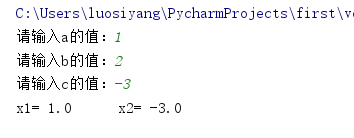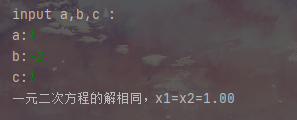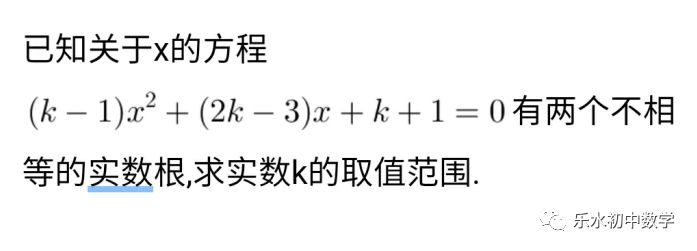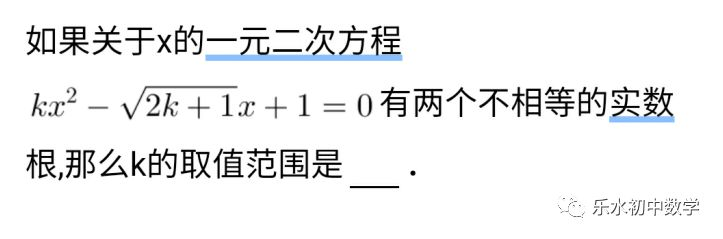• ## Python一元二次方程求根

万次阅读 多人点赞 2018-09-18 17:36:34
Python一元二次方程求根 1、任务简介 在之前的博客中我分享了使用Java进行一元二次方程求根的方法，在学习了Python之后我也想使用Python编写一个类似的程序，故在编写成功后将该任务分享出来。 2、任务代码 ...
Python一元二次方程求根
1、任务简介
在之前的博客中我分享了使用Java进行一元二次方程求根的方法，在学习了Python之后我也想使用Python编写一个类似的程序，故在编写成功后将该任务分享出来。

2、任务代码
学习过Java和Python的人都知道，Python的语法比Java简洁得多，并且目前已经广泛应用于爬虫开发、web开发、人工智能和机器学习等主流方向，是一种面向对象的语言，我学习Python的初衷就是为了学习爬虫，目前只是个小白，故只能给大家分享求根计算的程序，哈哈。
在Python中可以使用input来读取用户输入的信息，但是默认将用户输入的的信息保存为字符串的形式，所以需要强制类型转换为float的形式，这样在计算时才可以避免出现错误，在计算根时使用的语句和Java类似，但是math应全为小写，输出语句中的连接应该为逗号“，”。
程序代码如下：
import math
a = float(input("请输入a的值："))
b = float(input("请输入b的值："))
c = float(input("请输入c的值："))
x1=((-b+math.sqrt(b**2-4*a*c))/(2*a))
x2=((-b-math.sqrt(b**2-4*a*c))/(2*a))
print("x1=",x1,"\t","x2=",x2)

对比发现实现相同功能的Java程序有近19行，而Python程序仅有7行，实在是简洁得多。
运行结果如下图：还可以对程序进行改进，使它能判断是否有根等，具体代码如下：
import math
a = float(input("请输入a的值："))
b = float(input("请输入b的值："))
c = float(input("请输入c的值："))
if a != 0:
delta = b**2-4*a*c
if delta < 0:
print("无根")
elif delta == 0:
s = -b/(2*a)
print("唯一根x=",s)
else :
root = math.sqrt(delta)
x1 = (-b+root)/(2*a)
x2 = (-b-root)/(2*a)
print("x1=",x1,"\t","x2=",x2)

即便是加入这些功能，Python代码也仅有16行，故可以发现它相比Java的简洁性，改程序读者可自行验证，我就不发运行截图了。

3、总结
通过本博客分享了Python制作的一元二次方程求根小程序，大家可以对比之前Java编写该程序的博客《使用Java实现一元二次方程求根计算器》，可以发现两种语言之间部分语法是相通的，这也为我们的学习提供了方便。


展开全文• 其中，方程根的解法更是一元二次方程的重中之重，下面就给大家分析一下一元二次方程在初中学习中常见的方程的解法：求解一元二次方程求解一元二次方程方程常见的有三种方法：（1）公式法：将一元二次方程化为一般...
一元二次方程在整个数学的学习中是十分重要的，在初中来说它的地位更是高，不仅在中考数学中占有很大的比例，还在实际中也有很广泛的运用。其中，方程根的解法更是一元二次方程的重中之重，下面就给大家分析一下一元二次方程在初中学习中常见的方程的解法：求解一元二次方程求解一元二次方程方程常见的有三种方法：（1）公式法：将一元二次方程化为一般形式,然后利用求根公式 ,,() 当时，一元二次方程有两个不相等的实数根；当时，一元二次方程有两个相等的实数根；当时，一元二次方程没有实数根；例1用公式法求解方程的根。解：化简得>0                 ∴方程有两个不相等的实数根，利用公式得=∴或者公式法对任何一个一元二次方程都成立，时比较常用得求解一元二次方程的解的方法。（2）配方法：将一元二次方程化为的形式当p>0时，方程有两个不相等的实数根；当p<0时，方程没有实数根；当p=0时，方程有两个相等的实数根。（利用0划分是因为中，时,才有意义，P<0时，没有意义）例2.用配方法求解方程的解解：化简得进一步化简得∴两边同时开方得∴或者是注意：在配方时我们常将二次项得系数化为1，然后加上一次项系数得一半的平方，再减去一次项系数得一半的平方，将常数项合并，然后将常数项移到等式右边，等式左边即为完全平方式，最后等式两边同时开方就可得到方程的根。（数学表示法：可化简为,进一步化简为(3)因式分解法：将一元二次方程化简为左边为两个一次因式的乘积，右边等于0.我们知道如果两个因式乘积等于0，那么因式中任何一个为0；反之，如果两个因式中任何一个为0，那么他们的乘积也等于0.用数学符号表示为例3.用因式分解法求解方程解：化简得进一步化简得到(二重跟即方程有两个相等的根2)(4)韦达定理（即根与系数的关系）:方程()的两根为x和y,则有,例4.用跟与系数的关系求解方程解：化简得设方程两根为x,y,则      根据韦达定理可得x+y=-4/3，xy=1/3      由x+y=－4/3得x=－4/3－y        得y(－4/3－y)=1/3       得y=-1或y=－1/3       ∴方程的解为x=－1或x=－1/3总结：上面四种方法是我们求解一元二次方程时常用的方法，其中前三种是求解时最常见的，韦达定理在求根中并不常见，但在后面求解一元二次方程系数时是最常见的方法，因此，就算课本上韦达定理这一节带着*号，老师仍然还要着重强调的原因。今天，关于一元二次方程的题型就给大家分享这么多，明天将继续为大家分享一元二次方程常见的题型。
展开全文• 求根公式解一元二次方程 import math def quadratic(a, b, c): if a == 0: if b == 0: if c == 0: print('方程有任意解') else: print('方程无解') else: x = -c / b print('方程有解：x=%.2f'
用求根公式解一元二次方程
import math

if a == 0:
if b == 0:
if c == 0:
print('方程有任意解')
else:
print('方程无解')
else:
x = -c / b
print('方程有解：x=%.2f' % x)
else:
q = b * b - 4 * a * c
if q > 0:
x1 = (-b + math.sqrt(q)) / a / 2
x2 = (-b - math.sqrt(q)) / a / 2
print("一元二次方程的解为x1=%.2f，x2=%.2f" % (x1, x2))
return ()
elif q == 0:
x1 = -b / a / 2
x2 = x1
print("一元二次方程的解相同，x1=x2=%.2f" % (x1))
return x1, x2
else:
pass
print("一元二次方程无解")
return ()

print('input a,b,c :')
a = float(input('a:'))
b = float(input('b:'))
c = float(input('c:'))


效果图：一起学习python，小白指导，教学分享记得私信我


展开全文• 利用C语言判断一元二次方程的情况，并且一元二次方程
• 知识点8一元二次方程根的判别式的应用【题目预览】【视频讲解】【分析点评】此题考查一元二次方程根的判别式与一元二次方程根的情况，当判别式的值大于0时，方程有两个不相等的实数根，当判别式的值等于0时，方程有...知识点8 一元二次方程根的判别式的应用【题目预览】【视频讲解】【分析点评】此题考查一元二次方程根的判别式与一元二次方程根的情况，当判别式的值大于0时，方程有两个不相等的实数根，当判别式的值等于0时，方程有两个相等的实数根，当判别式的值小于0时，方程没有实数根。【举一反三】知识点1 一元二次方程的定义知识点2 一元二次方程的解的概念知识点3 用因式分解法解一元二次方程知识点4 用配方法解一元二次方程知识点5 用公式法解一元二次方程知识点6 用换元法解一元二次方程知识点7 一元二次方程根的判别式知识点8 一元二次方程根的判别式的应用知识点9 几何与方程相结合的问题知识点10 增长率问题知识点11 “每每”型经济问题知识点12 方案设计题知识点13 几何图形的面积问题知识点14 动态问题知识点15 最大值或最小值问题知识点16 一元二次方程根与系数的关系的应用知识点17 含有隐含条件的代数式值的问题知识点18 二次函数的定义知识点19 二次函数的图象知识点20 二次函数图象特征与系数之间的关系知识点21 用待定系数法求二次函数解析式知识点22 二次函数图象的性质知识点23 二次函数图象上点的坐标特征知识点24 二次函数与一元二次方程之间的转换知识点25 抛物线的轴对称变换知识点26 二次函数的应用——球类问题知识点27 二次函数的应用——拱桥问题知识点28 二次函数的应用——动态问题知识点29 二次函数的应用——决策问题知识点30 二次函数的应用——销售问题知识点31 二次函数的应用——分段函数问题知识点32 二次函数的应用——探索性问题知识点33 旋转变换的概念知识点34 旋转变换的性质知识点35 旋转变换中的角度、周长、面积的计算知识点36 中心对称变换知识点37 中心对称图案的设计知识点38 转化思想解定值问题知识点39 垂径定理的应用知识点40 圆心角与圆周角之间的关系知识点41 有关弦、半径、弦心距之间的问题知识点42 圆的内接四边形知识点43 分类讨论的数学思想知识点44 最短距离问题知识点45 点与圆的位置关系知识点46 直线与圆的位置关系知识点47 圆的切线的判定方法(1)知识点48 圆的切线的判定方法(2)知识点49 圆的切线的性质知识点50 切线长定理的应用知识点51 正多边形和圆知识点52 弧长的计算知识点53 扇形的面积问题知识点54 弓形的面积问题知识点55 圆锥的制作问题知识点56 圆锥和圆柱的组合问题知识点57 事件的可能性知识点58 利用频率估计概率的问题知识点59 事件的概率的方法(枚举法)知识点60 求事件的概率的方法(树状图法)知识点61 求事件的概率的方法(列表法)知识点62 概率的应用(图形变换型)知识点63 概率的应用(方程结合型)知识点64 概率的应用(函数结合型)知识点65 游戏公平性知识点66 反比例函数的概念知识点67 实际问题中的反比例函数知识点68 反比例函数的图象知识点69 反比例函数图象的性质知识点70 待定系数法求反比例函数的解析式知识点71 反比例函数与一次函数图象的交点问题知识点72 函数与方程组之间的转化知识点73 函数与不等式之间的转化知识点74 反比例函数中的力学问题知识点75 反比例函数中的动态问题知识点76 分段函数问题知识点77 数形结合解决反比例函数中的问题知识点78 一题多解在反比例函数中的运用知识点79 函数的猜想与验证知识点80 比例的基本性质知识点81 黄金分割点知识点82 相似多边形的性质知识点83 平行线分线段成比例定理的应用知识点84 相似三角形的判定方法(1)知识点85 相似三角形的判定方法(2)知识点86 探索性问题知识点87 应用相似三角形的性质解决线段问题知识点88 运用相似三角形的性质解决面积问题知识点89 相似在测量中的应用知识点90 相似中的探索问题知识点91 图形的位似知识点92 相似三角形中的动态问题知识点93 相似三角形的应用知识点94 三角函数的概念知识点95 特殊角的三角函数值的计算知识点96 含有三角函数值的代数式求值问题知识点97 根据三角函数值判断三角形的形状知识点98 构造直角三角形解决角度问题知识点99 三角函数与一元二次方程知识点100 坡度问题知识点101 探究航海问题知识点102 仰角与俯角的应用问题知识点103 构造直角三角形解决实际问题知识点104 平行投影的应用知识点105 中心投影的应用知识点106 物体的三视图知识点107 根据三视图求几何体的表面积知识点108 由物体的三视图探索立体图形的构成知识点108 圆锥的侧面展开图


展开全文• 本题目要求读入3个一元二次方程的系数a,b,c,出该方程的（也可能有复数）。 解题提示：Python的 math模块 中 sqrt 函数负数平方根会报错， 因为math 模块中 sqrt 函数只能进行浮点数的运算。我们知道负数的...
• 本文使用Python实现一元二次方程求根公式，主要演示运算符和几个内置函数的用法，封面图片与本文内容无关。def root(a, b, c, highmiddle=True): #首...人工智能 机器学习 js tapestry
• python计算一元二次方程 小编也是一个小白，，只因突然想起写个求解方程，所以写了一个求解一元二次方程的代码。 发现使用python写求解方程要比c语言简单。。但是学艺不精，，勿喷 以下代码能够实现有解的...
• 主要介绍了Python编程实现数学运算求一元二次方程的实算法,涉及Python数学运算求解方程的相关实现技巧,需要的朋友可以参考下
• 本文实例讲述了Python编程实现数学运算求一元二次方程的实算法。分享给大家供大家参考，具体如下：问题：请定义一个函数quadratic(a, b, c)，接收3个参数，返回一元二次方程：ax² + bx + c = 0的两个解。实现代码...
• python一元二次方程解 import math def quadratic(a,b, c): jie=() if a == 0: print("a为分母，不能为0") elif (b ** 2 - 4 * a * c) &lt; 0: jie=("无解") return (jie) print...
• 一元二次方程求根程序Here you will get simple C++ program to find roots of quadratic equation ax2+bx+c=0. 在这里，您将获得简单的C ++程序，以找到二次方程ax 2 + bx + c = 0的。 #include<iostream>...c++ java linux
• python一元二次方程系数求解 提示：以下是本篇文章正文内容，下面案例可供参考 代码如下 代码如下（示例）： #一元二次方程系数求解 import math #引入数学函数 a,b,c = eval(input()) #定义变量 d = b ** 2 - 4 *...机器学习
• Python3 实例二次方程式 # Filename : test.py # author by : www.runoob.com # 二次方程式 ax**2 + bx + c = 0 # a、b、c 用户提供 # 导入 cmath(复杂数学运算) 模块 import cmath a = float(input('输入 a:...
• 本文实例讲述了Python编程实现数学运算求一元二次方程的实算法。分享给大家供大家参考，具体如下：问题：请定义一个函数quadratic(a, b, c)，接收3个参数，返回一元二次方程：ax² + bx + c = 0的两个解。实现代码...
• Here you will get C program to find roots of quadratic equationax2+bx+c=0 在这里，您将获得C程序来查找二次方程ax 2 + bx + c = 0的 #include<stdio.h> #include<math.h> int main() { float ...算法 linux c++
• 11、定义一个函数 “quadratic(a,b,c)”，接收三个参数，返回一元二次方程: ax² + bx + c = 0 的两个解。（提示：计算平方根可以调用math.sqrt()函数） 以下是我写的代码 import math def quadratic(a, b, c): ...
• 萌新练手 import math def qua(a, b, c): a1 = -b - math.sqrt(b * b - 4 * a * c) ...a1, b1, c1 = map(int, input("☆一元二次方程计算器☆，请输入a,b,c系数，以空格隔开！\n☆输入0 0 0可退出程序☆\n\n").split()有问必答
• 题目：请定义一个函数 ’quadratic(a,b,c)‘，接收三个参数，返回一元二次方程:ax2 + bx + c = 0的两个解。（提示：计算平方根可以调用math.sqrt()函数）import mathdef quadratic(a, b, c):if not isinstance(a, ...
• Python实现求解一元二次方程的方法示例本文实例讲述了Python实现求解一元二次方程的方法。分享给大家供大家参考，具体如下：1. 引入math包2. 定义返回的对象3. 判断b*b-4ac的大小具体计算代码如下：# -*- coding:utf...
• from math import * print("根据a*x^2+b*x+c=0,在a≠0的情况下，有两个... print("该方程没有实") else: x1=(-b+sqrt(b**2-4*a*c))/(2*a) x2=(-b-sqrt(b**2-4*a*c))/(2*a) print("x1=%6.2f\nx2=%6.2f"%(x1,x2))python...

# python一元二次方程求根python 订阅